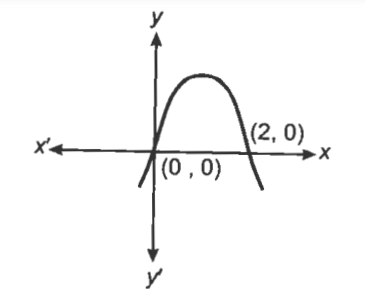26 views

The area of the region bounded by the curve y = 2x – x2 and x – axis is

A)$$\frac{2}{3} sq.units$$

B) $$\frac{4}{3}sq.units$$

C) $$\frac{5}{3} sq.units$$

D)$$\frac{8}{3} sq.units$$

| 26 views

Correct answer is $$\frac{4}{3}sq.units$$

Explaination::Given curve is $$y =2 x-x^{2}$$  or $$(x-1)^{2} =-(y-1)$$

The curve cut the x -axis at (0,0) and (2,0) .

$$\therefore \ Required\ area =\int_{0}^{2} y d x$$

$$=\int_{0}^{2}\left(2 x-x^{2}\right) d x=\left[x^{2}-\frac{x^{3}}{3}\right]_{0}^{2}$$

$$=4-\frac{8}{3}=\frac{4}{3} sq \ unit$$

by Expert (10.9k points)